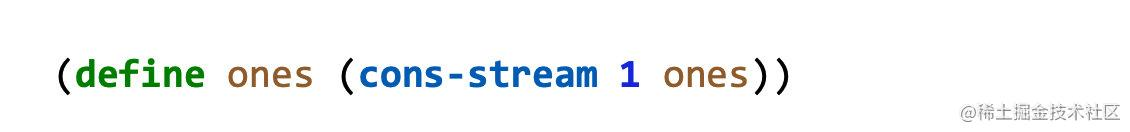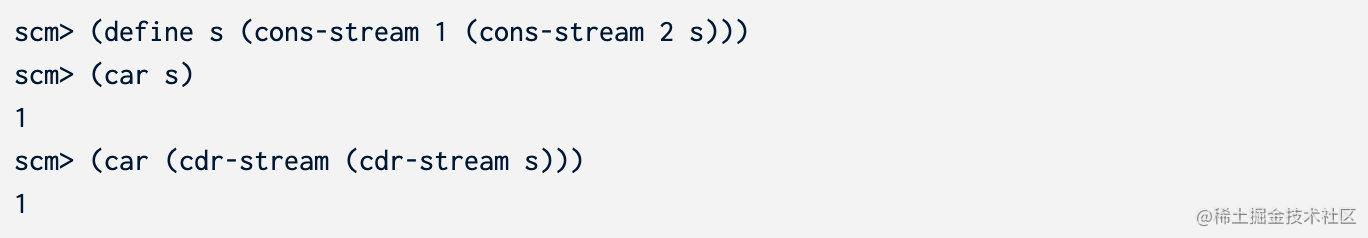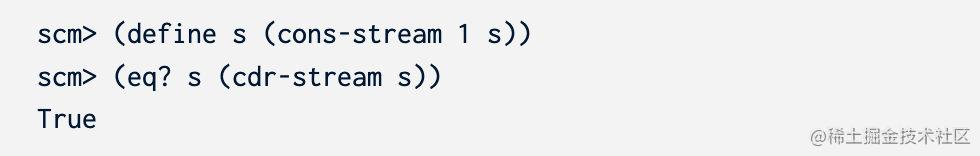# 日拱一卒，伯克利CS61A，用Scheme判断链表是否有环Github### Q1: Find

predicate 是一个接收一个参数的函数，如果传入的数据不合法返回`False`，否则返回`True`。如果找不到合法的元素，返回`False`

``````python3 ok -q find

#### 答案

``````(define (find s predicate)
(if (null? s) False
(if (predicate (car s)) (car s)
(find (cdr-stream s) predicate)
)
)
)

### Q2: Scale Stream

``````python3 ok -q scale-stream

#### 答案

``````(define (scale-stream s k)
(if (null? s) nil
(cons-stream (* (car s) k) (scale-stream (cdr-stream s) k))
)
)

### Q3: Cycles`eq?`函数对你也许很有用，它可以判断两个stream是否相同``````python3 ok -q has-cycle

#### 答案

``````(define (has-cycle s)
(define (in? mem s)
(cond
((null? mem) False)
((eq? (car mem) s) True)
(else (in? (cdr mem) s))
)
)
(define (has-cycle-helper mem s)
(cond
((null? s) False)
((in? mem s) True)
(else (has-cycle-helper (cons s mem) (cdr-stream s)))
)
)
(has-cycle-helper nil s)
)

### 附加题

#### 答案

``````(define (has-cycle-constant s)
(define (has-cycle-helper fast slow)
(cond
((null? fast) False)
((null? (cdr-stream fast)) False)
((null? slow) False)
((eq? fast slow) True)
(else (has-cycle-helper (cdr-stream (cdr-stream fast)) (cdr-stream slow)))
)
)
(cond
((null? s) False)
((null? (cdr-stream s)) False)
(else (has-cycle-helper (cdr-stream (cdr-stream s)) (cdr-stream s)))
)
)